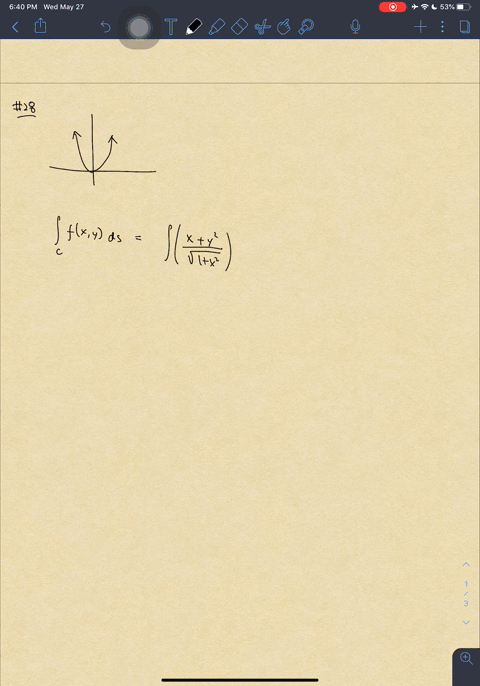🎉 The Study-to-Win Winning Ticket number has been announced! Go to your Tickets dashboard to see if you won! 🎉View Winning Ticket## Discussion

You must be signed in to discuss.

## Video Transcript

Okay, folks. So in this video, we're going to take a look at problem number 27 which is a problem where were given a function than used to be integrated along a curve and were given to function for why, as a function of X along the curve, everywhere why can be written in terms of eggs on and that function is X squared over two. So we're going to plug in this export over to this expression into the function. Did the function f um and I'm going to skip over a few intermediate steps here. So we have this integral along. See, f x Y d s. This is the usual line. Integral, you know, And you know the drill DS can be rewritten in terms of in terms of d, X and F. I'm going to plant in why? But not why itself. But why the function of X, which is X squared over two. So, after skipping through a few intermediate steps here we have between zero and two x cubed over X squared times two and that's for F. And the S is simply X squared plus one dx. And this is schooling. But this is gonna be simplified into twice. Um, X times one plus x squared the X. Now, I am going to define a new variable here. Ah, variable called you. I'm going to find that as x squared plus one. So D you is simply too x dx. This is called you substitution, which I'm sure a lot of you have heard of. So now I'm gonna plug in this expression back into this integral, okay? And that integral simply becomes twice of route you did you over to and this is gonna be evaluated into ah u to the power of 3/2 over three have between one and five. Now, if you're curious where I got this one and five from, I simply plucked in the old integration limits, which is the or into. I pluck in those two numbers into this expression for you, which is in terms of X. So if you plug in zero year, you get zero squared plus one, which is one, and you get five if you plug in two. So two's great plus one is five. So that's that's how you get the new integration limits. And if you evaluate this thing. You're going to get to third five to the power of 3/2 minus one. And this is simply this is a very simple algebra problem. As soon as you realize that as soon as you set out, as soon as you set up the integral and integration limits and all those kind of things after you set those things up, you're going to have a very simple algebra problem, which you can just solve and evaluate and crank out the numbers. And you're going to arrive at this number right here, which is the answer for problem problem 27. That's it for this video. Thank you. Bye.